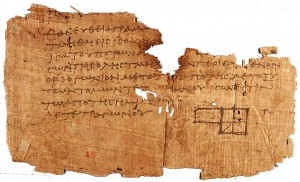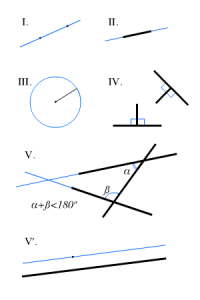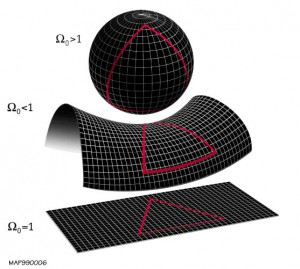Select PageAround the second century BC, a man from Alexandria and his disciples wrote one of the books that would change history, “The Elements”, in which the properties of lines, planes, circles, spheres, triangles and cones are studied … leaving only five postulates:

I. “To draw a straight line from any point to any point.”

II. “To produce [extend] a finite straight line continuously in a straight line.”

III. “To describe a circle with any centre and distance [radius].”

IV. “All right angles are equal to one another.”

V. The parallel postulate: “if a straight line falling on two straight lines makes the interior angles on the same side less than two right angles, the two straight lines, if produced indefinitely, meet on that side, where the angles are inferior to the two right angles.”These principles define the Euclidean space, the space in which we live or we think we live. In this “world”, the sum of the angles of a triangle is equal to 180º, however, what if this is not fulfilled?, wether the result of that sum gave a slightly different result, would bring down the pillars of the Universe, and many of the basic ideas that we consider to be untrue. So, the next time we make calculations, beware, the universe’s future is in our hands.

Many years after “The elements”, a world citizen shook the foundations of society. The space is not euclidean, the angles of a triangle not always sum 180º, then, a mathematical rampant horror travelled the world. General relativity introduced curved space, which is the amount by which a geometric object in a Euclidean space deviates from being flat.

We can build three spaces:

• Euclidean space.
• Bolyai-Lobachevsky hyperbolic open space: where lines “curve away” from each other, increasing in distance as one moves further from the points of intersection with the common perpendicular; these lines are often called ultraparallels.
• Elliptical closed Riemann space: the lines “curve toward” each other and intersect.To determine the morphology of the Universe, we can simplify their curvature in flat, positive and negative. According to the FLRW model, exact equations general relativity solution, the universe is a moving space, homogeneous and isotropic (flat).

In spherical local geometry (positive), on a fixed point does not necessarily pass any parallel and the sum of the interior angles of triangles is greater than 180 °. This universe is generally associated with a finite universe which has a point of temporary space ending.

In the hyperbolic (negative) local geometry may pass over a point which two parallel and the sum of the interior angles of the triangles is less than 180 °. The ultimate fate of an open universe: it continues to expand forever, ending in a cold death of the universe.

Rubén Suárez